Short-term Earthquake Prediction Based on Seismic Precursory Electric  Signals Recorded on Ground Surface.

“What today seems impossible, is tomorrow’s reality”

Dinos

"STRANGE ATTRACTOR LIKE" ELECTRIC EARTHQUAKE PRECURSORS

SYNTHETIC DATA

The application of the "strange attractor" EQ electrical precursor has shown that it takes the form of a hyperbola, ellipse or closely to circle, in cases when the signal to noise ratio is quite large.

In this part of the presentation "clean" (completely free of any noise) signals will be used in order to check the physical validity of the specific EQ electric precursor.  The used physical set-up  basically consists of two distant  monitoring sites.

Generation of hyperbolas.

This is demonstrated in the following Fig. (8).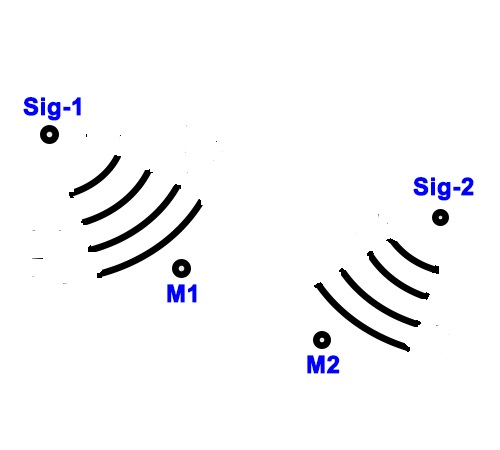Fig.8. Monitoring sites M1,2 register signals generated at different locations Sig-1,2

The generated signals are uncorrelated. The application of the "strange attractor" method  results are shown in the following Fig. (9).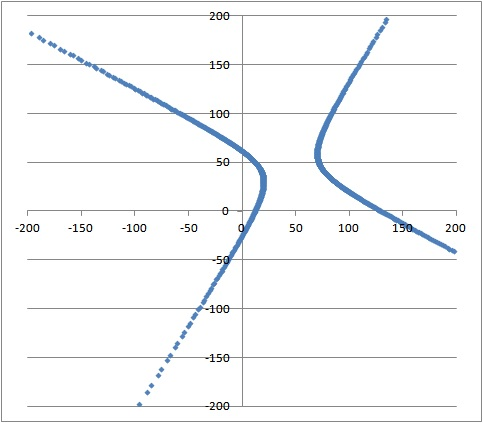Fig. 9. Hyperbolas generated by the application  of the "strange attractor" method on uncorrelated noise electrical signals.

Generation of circles.

In the physical model of Fig. (8) the two noise generation sources Sig-1,2 are replaced by a single signal source (EQ focal area). The generated  signal is registered by the two monitoring stations M1,2. The physical set-up of this version is shown in the following Fig. (10).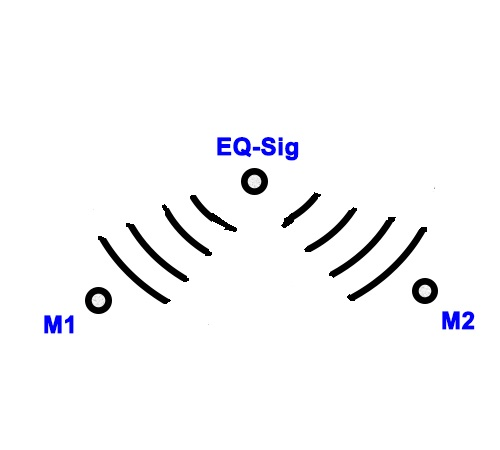Fig. (10). Monitoring sites M1,2 register signals generated at the EQ focal area  (EQ-Sig).

The generated signals are correlated. The application of the "strange attractor" method  results are shown in the following Fig. (11).Fig. (11). Circle created by the application of the "strange attractor" EQ electric precursor, on the same monochromatic EM signals induced by the EQ focal area at both monitoring sites M1,2.

Generation of  an ellipse.

The next step in this analysis is to transform a calculated circle into an actually observed ellipse. At this point you have to remember that both signal and noise are of the same frequency. Therefore, the monochromatic EQ EM signal is interfered by a monochromatic noise of the same frequency as the EQ signal.

The application of the "strange attractor" method  simultaneously on both signals, results into    the following Fig. (12).Fig. (12). Ellipse created by the application of "strange attractor" methodology on  both monochromatic EQ electric precursory signal and noise.

At this point one could ask: How do you know that  a circle is generated always from your model and no any other shape?

There are three (3) ways to prove it.

The first one is  a tedious and lengthy mathematical  transformations of the physical model equations aiming to show that each point (x,y) generated from the model is placed at a fixed distance (radius) from a fixed point which is the center of the circle. I advise you not to do it.

The second is to test it statistically. In this case there is the problem of the "statistical significance" of the No of examples used which in turn leads to an endless debate on the topic.

The third one, being the most elegant,  beautiful and simple too, is based on the physical processes which take place in the model, and to give you a hint, some high-school geometry (angles in circles properties) is required.

During this work we had an exceptional idea. A virtual (hypothetical) network, composed of three monitoring sites and a hypothetical EQ, to be considered and the resulting circles to be placed in the same graph for comparison reasons. The following virtual specific cases have been considered:

A. Monitoring sites M1, M2, M3 and hypothetical EQ-1

B. Monitoring sites M1, M2, M3 and hypothetical EQ-2

C. Monitoring sites M4, M5, M6 and hypothetical EQ-2

In detail:

A. The monitoring sites M1, M2, M3 and the hypothetical EQ-1 coordinates are:

 COR X Y M1 = 30 20 M2 = 60 50 M3 = 80 90 EQ-1 45 75

Circles are formed in pairs of monitoring sites (M1-M2, M1-M-3, M2-M3). The corresponding results are shown in Fig. (13).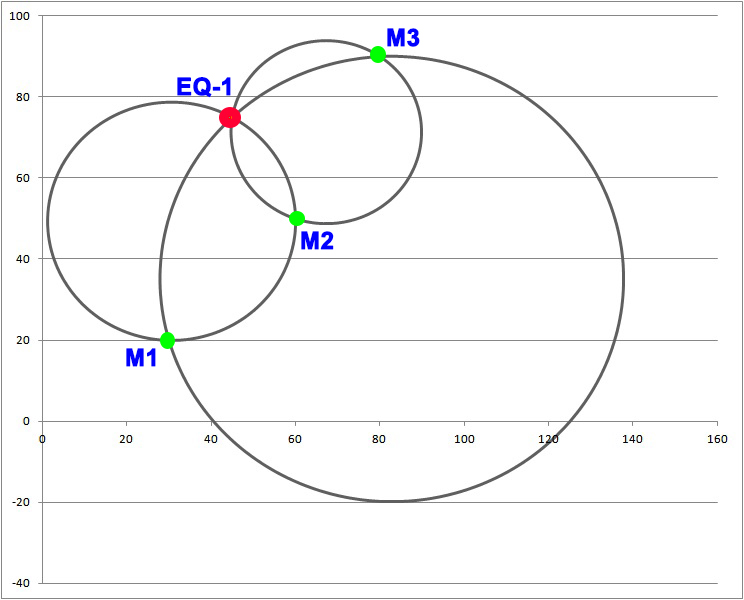Fig. 13. Circles generated by the application the "strange attractor" electric precursor on  signals generated by EQ-1. The double circles intersections indicate the monitoring sites while the triple one indicates the hypothetical EQ-1 location (compare its theoretical and resulted coordinates).

B. The monitoring sites M1, M2, M3 and the hypothetical EQ-2 coordinates are:

 COR X Y M1 = 30 20 M2 = 60 50 M3 = 80 90 EQ-2 120 10

Circles are formed in pairs of monitoring sites (M1-M2, M1-M-3, M2-M3). The corresponding results are shown in Fig. (14).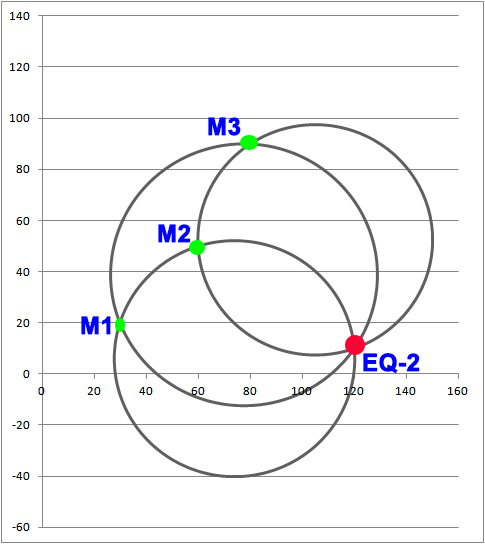Fig. 14. Circles generated by the application of the "strange attractor" electric precursor on the signals generated by EQ-2. The double circles intersections indicate the monitoring sites while the triple one indicates the hypothetical EQ-2 location (compare its theoretical and resulted coordinates).

C. The monitoring sites M4, M5, M6 and the hypothetical EQ-2 coordinates are:

 COR X Y M4 = 10 90 M5 = 15 20 M6 = 110 100 EQ-2 120 10

Circles are formed in pairs of monitoring sites (M4-M5, M4-M-6, M5-M6). The corresponding results are shown in Fig. (15).Fig. 15. Circles generated by the application of the "strange attractor" electric precursor on signals generated by EQ-2. The double circles intersections indicate the monitoring sites while the triple one indicates the hypothetical EQ-2 location (compare its theoretical and resulted coordinates).

In all three cases A, B and C, the epicenter of the hypothetical EQ is determined with high accuracy. The next step is to check the validity of the mathematical analysis of the virtual model by using Inversion  (Example-1)  methodologies.